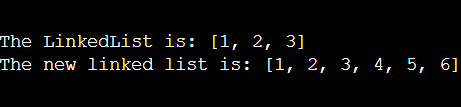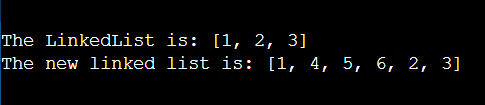## Introduction:

LinkedList is a linear data structure that is used to store the data. There is a very famous and useful method for LinkedList that is addAll() method. This method is basically used to add data from any Collection to our LinkedList.

There are two ways to apply this method.

Let us discuss each of them:-

Using the above way we can add the data of any Collection at the end of the LinkedList, in the same manner, they were in the Collections.

The above method returns true if at least one element is appended to the LinkedList.

Refer to the below code for more understanding.

Output:## 2) addAll(int index, Collection X):

Using the above way we can add the data of any Collection at the particular index of the LinkedList, in the same manner, they were in the Collections.

Syntax: boolean addAll(int index, Collection X)

The above method returns true if at least one element is appended to the LinkedList.

Refer to the below code for more understanding.

Output:Time Complexity: The time complexity for both of the above functions is O(N)(where N is the total number of elements in the Collection’s list to be added).

Space Complexity: Both of the above functions does not use any auxiliary space internally. Hence, the space complexity is O(1).

## FAQs:

1. What are the two types of addAll() method we can use?
The two types of addAll method are addAll(Collection X) used to add all the elements of a Collection at the end of the LinkedList. The other is addAll(int index, Collection X) that is used to add the data of any Collection at the particular index of the LinkedList.
2. What is the time complexity for both functions?
The time complexity for both of the above functions is O(N)(where N is the total number of elements in the Collection’s list to be added).

## Key Takeaways:

In this blog, we have covered the following things:

2. Then we discussed the two types of addAll method in Java.

Until then, All the best for your future endeavors, and Keep Coding.